• 密度图 给定一个数据集，需要观察这些样本的分布情况，往往我们会采用直方图的方法来进行直观的展现。该方法简单，容易计算，但是直方图存在着如下的两大问题： 绘制直方图时，需要确定分组问题，如果分组不同，...
• r语言密度图You can create a density plot in R using ggplot2. For plotting using ggplot2, you have to use the function geom_density(). Let’s see how it works in this tutorial. 您可以使用ggplot2在R...

r语言中 密度图

You can create a density plot in R using ggplot2. For plotting using ggplot2, you have to use the function geom_density(). Let’s see how it works in this tutorial.
您可以使用ggplot2在R中创建密度图。 要使用ggplot2进行绘图，必须使用函数geom_density（）。 让我们看看它在本教程中是如何工作的。
什么是密度图？ (What is a Density plot?)
Density plots, also known as Kernel density plots, they’re are used to understand the distribution of data. It is considered as an effective way to present the variable distribution over the given time period. The density plot’s peak gives the data of concentrated values over the time period.
密度图，也称为内核密度图 ，用于了解数据的分布。 被认为是呈现给定时间段内变量分布的有效方法。 密度图的峰值给出了一段时间内的集中值数据。

You can either create a density plot in basic R or by using ggplot as mentioned above.
您可以在基本R中创建密度图，也可以如上所述使用ggplot创建密度图。

密度图相对于直方图的优势 (Advantages of Density plots over Histograms)
Density plots are better than histograms, as they can determine the distribution shape effectively. 密度图比直方图更好，因为它们可以有效地确定分布形状。 Unlike histograms, density plots are not affected by bins. 与直方图不同，密度图不受容器影响。 It gives a clear visualization of the data distribution over a time period. 它可以清晰显示一段时间内的数据分布。
There are many types of density plots are available to plot in R. All of them are used based on various problems and requirements.
在R中可以绘制多种类型的密度图。根据各种问题和要求使用所有密度图。
Let’s roll into the topics and we can plot all types of density plots in R
让我们进入主题，我们可以在R中绘制所有类型的密度图

在R中使用ggplot2的基本密度图 (Basic density plot using ggplot2 in R)
In this section we are creating a basic density plot using ggplot2 in R. For this purpose, we will import a pricing data file. After that, we will plot the density plot for the values present in that file.
在本节中，我们将使用R中的ggplot2创建基本密度图。为此，我们将导入定价数据文件。 之后，我们将绘制该文件中存在的值的密度图。

您可以从此链接下载本教程中使用的示例文件。  来源 ： 虹膜数据集 ， Google Play商店应用数据集
Execute the below code to create a simple density plot in Rstudio.
执行以下代码以在Rstudio中创建一个简单的密度图。

library(ggplot2)               #imports ggplot2
library(dplyr)                 #imports dplyr

#creating the density plot
data %>%
+     filter( Dollars<400 ) %>%
+     ggplot( aes(x=Dollars)) +
+     geom_density(fill="#4D9DDA", color="#4D9DDA", alpha=0.8)



Density Plot in R

Now we will try to add a title to our density plot. Execute the below code to create a density plot with a suitable title.
现在，我们将尝试为密度图添加标题。 执行以下代码以创建具有合适标题的密度图。

library(ggplot2)               #imports ggplot2
library(dplyr)                 #imports dplyr

#creating the density plot
data %>%
+     filter( Dollars<400 ) %>%
+     ggplot( aes(x=Dollars)) +
+     geom_density(fill="#4D9DDA", color="#4D9DDA", alpha=0.8)+
+    ggtitle("State wise population distribution in the USA")+theme_ipsum()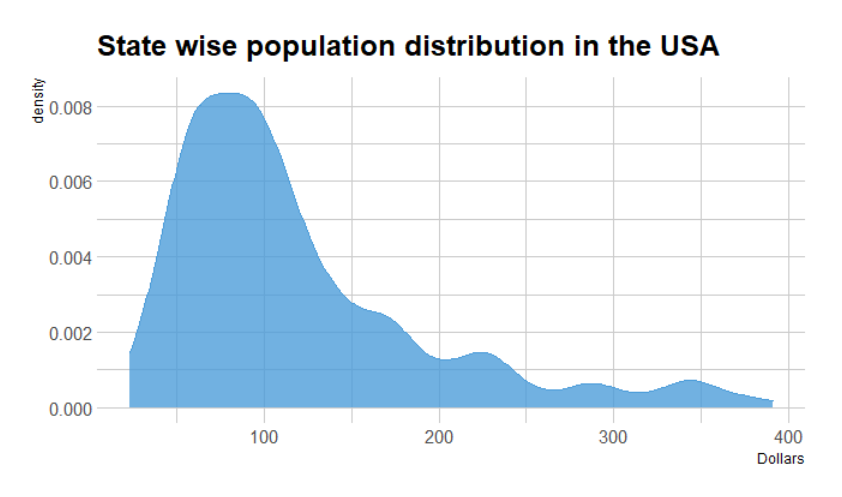hrbrtheme: hrbrthemes is an additional theme package in ggplot2 which mainly concentrates on typography in the plots.
hrbrtheme： hrbrthemes是ggplot2中的另一个主题包，主要集中于情节中的版式。

使用ggplot2在R中的镜像密度图 (The Mirror density plots in R using ggplot2)
As you know that the density plots are the representation of the distribution of the values. The mirror density plots are used to compare the 2 different plots.
如您所知，密度图是值分布的表示。 镜面密度图用于比较两个不同的图。
The exactly opposite or mirror plot of the values will make comparison very easy and efficient. For creating this mirror density plot using ggplot2, we use geom_density function.
值的完全相反或镜像绘制将使比较非常容易和有效。 为了使用ggplot2创建此镜像密度图，我们使用geom_density函数。
To plot the mirror density plot, execute the below code in Rstudio.
要绘制反射镜密度图，请在Rstudio中执行以下代码。

library(ggplot2)       #importing library ggplot2
library(hrbrthemes)    #importing library hrbrthemes

#sample data taken for plotting
data <- data.frame(data1 = rnorm(1000),data2 = rnorm(1000, mean=2))

p <- ggplot(data, aes(x=x))+

#top portion plot
geom_density( aes(x = data1, y = ..density..), fill="#D2CE12" ) +
geom_label( aes(x=6, y=0.20, label="data1"), color="#1EAEC2") +

#bottom portion plot
geom_density( aes(x = data2, y = -..density..), fill= "#66B32D") +
geom_label( aes(x=6, y=-0.20, label="data2"), color="#1EAEC2") +
theme_ipsum() +
xlab("x values")使用ggplot2在R中的多个密度图 (Multiple Density plots in R using ggplot2)
Multiple density plots: These are the plots that use multiple variables and multiple fills to create a graph, which shows the distribution of values.
多个密度图：这些图使用多个变量和多个填充来创建图形，以显示值的分布 。
In this section, we are going to create multiple density plots using ggplot2. In this plot, we are using the google play store data which is available in Kaggle.
We are plotting the graph using the Content Rating and Numbers data.
我们正在使用“ 内容分级和数字”数据绘制图表。

Execute the below code to create the multiple density plot in R studio.
执行以下代码以在R studio中创建多重密度图。

library(ggplot2)     #imports library ggplot2
library(hrbrthemes)  #imports library hrbrthemes
library(dplyr)       #imports the dplyr function
Library (tidyr)      #imports the tidyverse package
library(viridis)     #imports the library viridis

x1 <- ggplot(googleplaystore, aes(x=Number, group=Content.Rating, fill=Content.Rating))+
theme_ipsum()
x1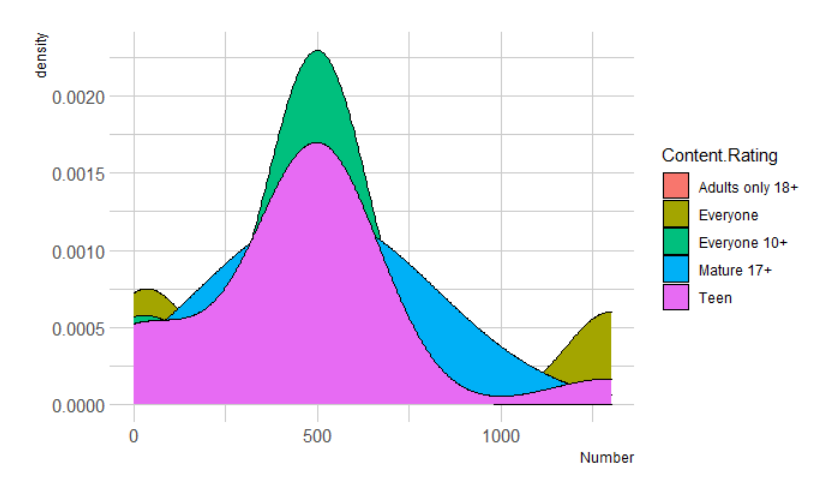使用facet_wrap（）函数创建小倍数 (Creating Small Multiples using facet_wrap() function )
This small multiples of the density plot will help us to understand the distribution of each variable. The individual plots will help us to compare the different variable distribution as they lie on the same axis.
密度图的这个小倍数将帮助我们理解每个变量的分布。 各个图将帮助我们比较位于同一轴上的不同变量分布。
For this purpose we are using facet_wrap() function.
为此，我们使用facet_wrap（）函数。
To create the small multiples of the density plot, execute the below code in Rstudio.
要创建密度图的较小倍数，请在Rstudio中执行以下代码。

theme_ipsum() +
facet_wrap(~Content.Rating) +    #creates the small multiples
theme(
legend.position="none",
panel.spacing = unit(0.2, "lines"),
axis.ticks.x=element_blank()
)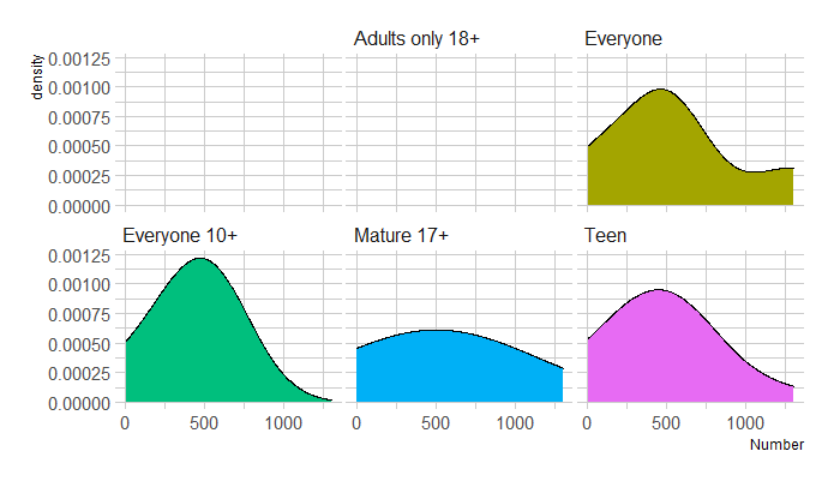使用ggplot2在R中的堆积密度图 (Stacked density plots in R using ggplot2)
The stacking density plot is the plot which shows the most frequent data for the given value. But the disadvantage of the stacked plot is that it does not clearly show the distribution of the data.
堆积密度图是显示给定值的最频繁数据的图。 但是堆积图的缺点是它不能清楚地显示数据的分布。
Here we are creating a stacked density plot using the google play store data.
Execute the below code to create the stacked density plot in R studio.
执行以下代码以在R studio中创建堆积密度图。

plt <- ggplot(data=readfile, aes(x=Number, group=Content.Rating, fill=Content.Rating)) +
theme_ipsum()

plt   #displays the plot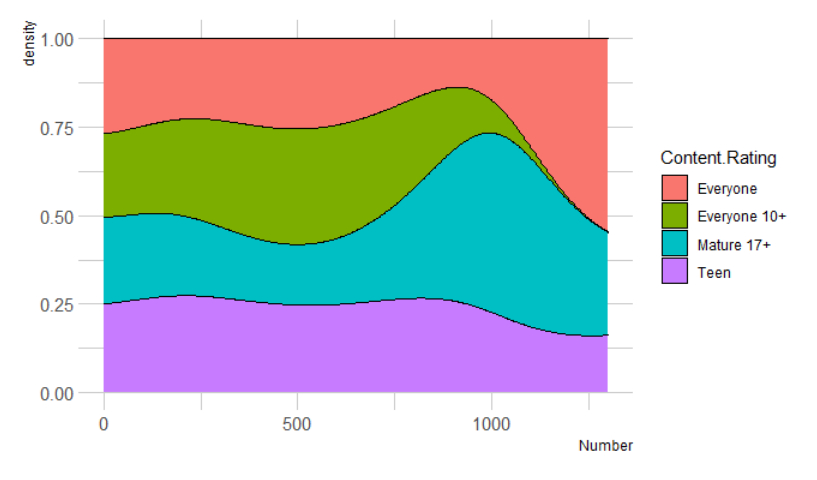使用ggplot2在R中的2D密度图 (2D Density plots in R using ggplot2)
R offers the function geom_density2d() to plot the two dimensional density plots. 2D graphs are visually appealing in nature and can communiacte the insights in an effective manner .
R提供函数geom_density2d（）来绘制二维密度图。 2D图形本质上在视觉上很吸引人，并且可以有效地交流见解。
For this purpose we are using the iris flower dataset which is available in the kaggle webiste. Lets plot the density plot for sepal length and with varibales.
为此，我们使用kaggle网站中提供的鸢尾花数据集。 让我们绘制出萼片长度和方差的密度图。
Execute the below code to create a 2D density plot in R studio.
执行以下代码以在R studio中创建2D密度图。

library(ggplot2)

#marks the x and y axis values
View(x)

#generated the 2D density plot
x+stat_density2d()+geom_point()

#create an appealing 2D plot
values+stat_density2d(aes(fill=..density..), geom='raster',contour=FALSE)
values+stat_density2d(aes(fill=..density..), geom='tile',contour=FALSE)+geom_point(color='white')

#shows the density points in the plot
densitypoints <- values+stat_density2d(aes(fill=..density..), geom='tile',contour=FALSE)+geom_point(color='white')

#creates the x and y labels
densitypoints+xlab('Sepal length')+ylab('Sepal width')


The iris flower data is shown below.
鸢尾花数据如下所示。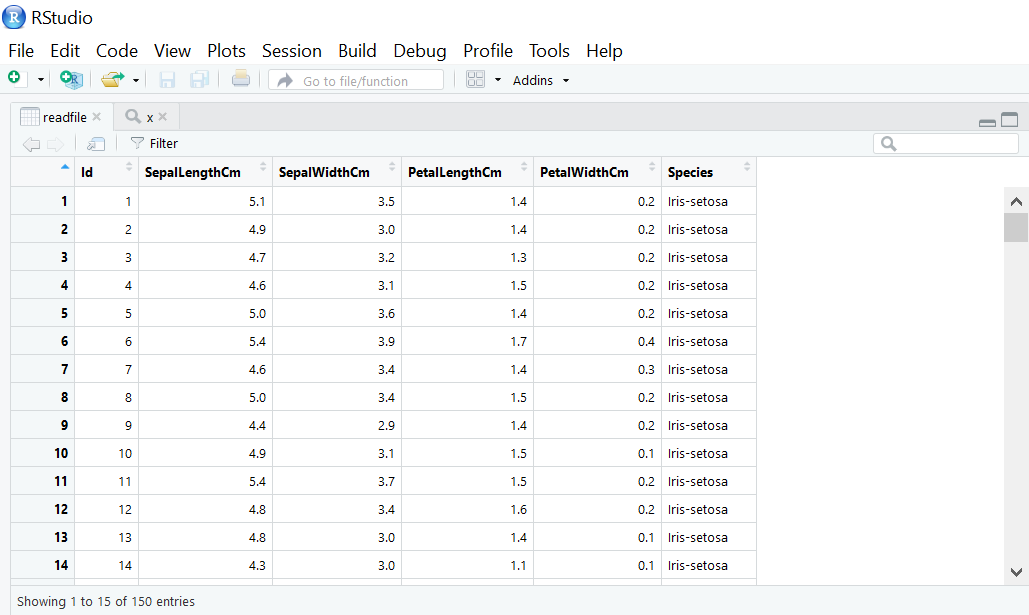The 2-Dimensional density plot of the values present in iris flower data.
鸢尾花数据中存在的值的二维密度图。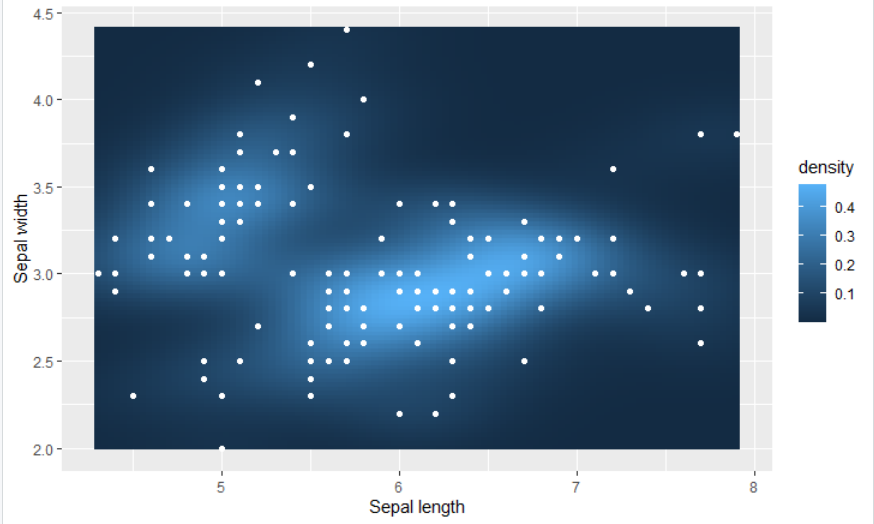结论 (Conclusion)
R is one of the best visualization heavy language. In this tutorial, we have gone through the density plots, its benefits and we have plotted the density plots using ggplot2.
R是最好的可视化繁重语言之一。 在本教程中，我们详细介绍了密度图及其优点，并使用ggplot2绘制了密度图。
There are many types of density plots in R. We have plotted basic density plots with x and y labels, mirror density plots, multi-density plots, stacked density plots and finally we have plotted the 2-dimensional density plot using the iris dataset.
R中有很多类型的密度图。我们已经绘制了带有x和y标签的 基本密度图 ， 镜面密度图 ， 多密度图，堆积密度图 ，最后我们使用虹膜数据集绘制了二维密度图 。
R offers great libraries and functions to create visually appealing graphs for any purpose. That’s all for now. Learn more…Practice more and stay connected for more R tutorials. Keep learning!!!
R提供了出色的库和函数 ，可以为任何目的创建吸引人的图形 。 目前为止就这样了。 了解更多…更多实践，并保持联系以获取更多R教程。 保持学习！！！

翻译自: https://www.journaldev.com/38367/density-plot-in-r

r语言中 密度图

展开全文• ## R语言作图——density plot(密度图)

万次阅读 多人点赞 2018-10-09 13:22:57
上次分享了小提琴曲线(violin plot)的作图方法,今天小仙同学给大家介绍一下如何用R画出漂亮的密度图(density plot)。 Step1. 绘图数据的准备 首先还是要把你想要绘图的数据调整成R语言可以识别的格式excel中保存成...
原创 黄小仙
上次分享了小提琴曲线(violin plot)的作图方法,今天小仙同学给大家介绍一下如何用R画出漂亮的密度图(density plot)。
Step1. 绘图数据的准备 首先还是要把你想要绘图的数据调整成R语言可以识别的格式，把excel保存成csv格式。 数据的格式如下图：一列表示一种变量，第一行是列名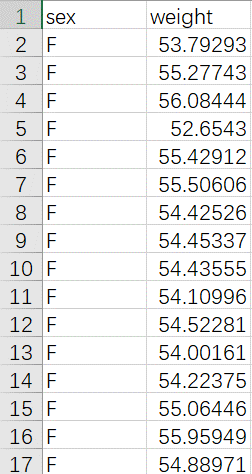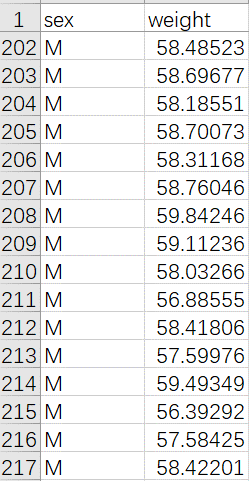Step3.绘图所需package的安装、调用 install.package(“ggplot2”) #注释：ggplot2是目前公认绘图很强的一个安装包，如果已经安装，这句就不需要啦 library(ggplot2) #注释：package使用之前需要调用(每次用前都要调用哦)
Step4绘图 p<-ggplot(data, aes(x = weight)) #注释：x轴表示weight，y轴表示频率就不需要指定啦 p + geom_density(color = “black”, fill = “gray”) #注释：color是线条的颜色参数，fill表示填充颜色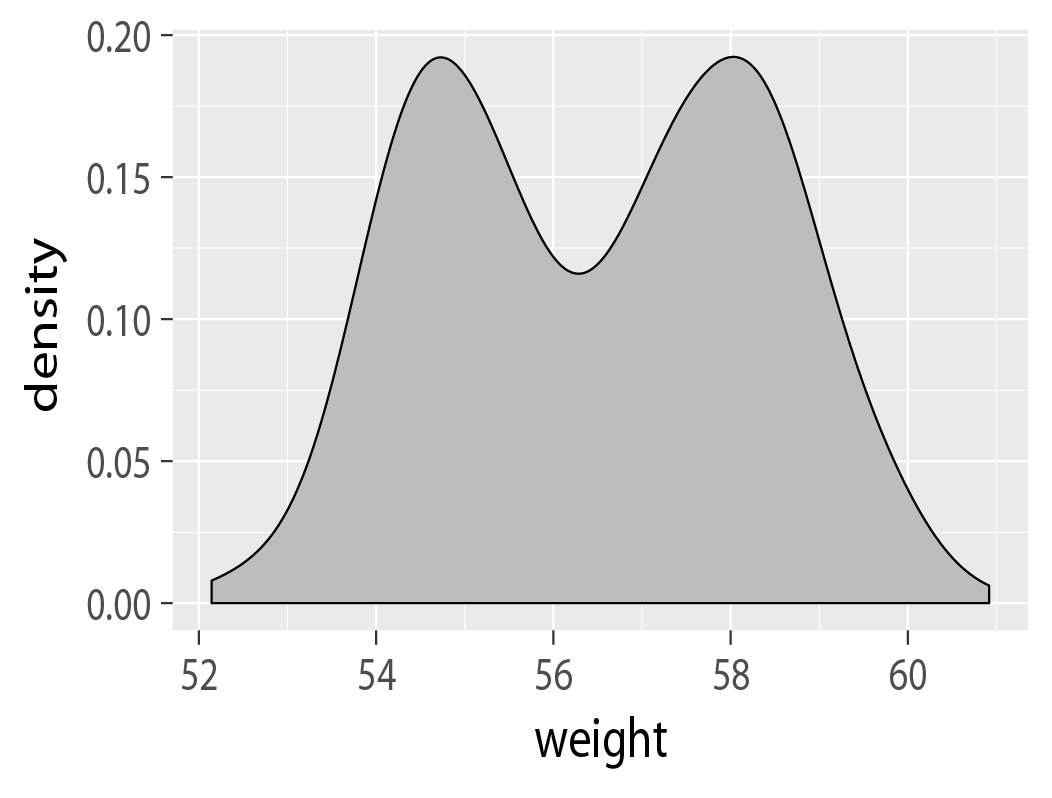Step5.美化 p + geom_density(aes(color = sex)) #注释:按照性别不同组改变线条颜色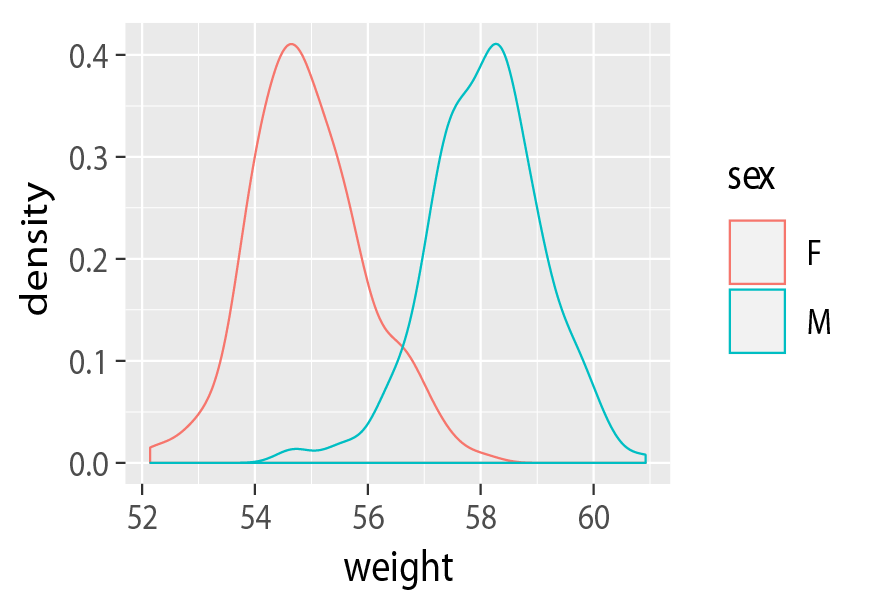p + geom_density(aes(fill = sex), alpha=0.4) #注释:按照性别不同组改变填充颜色，alpha表示调整透明度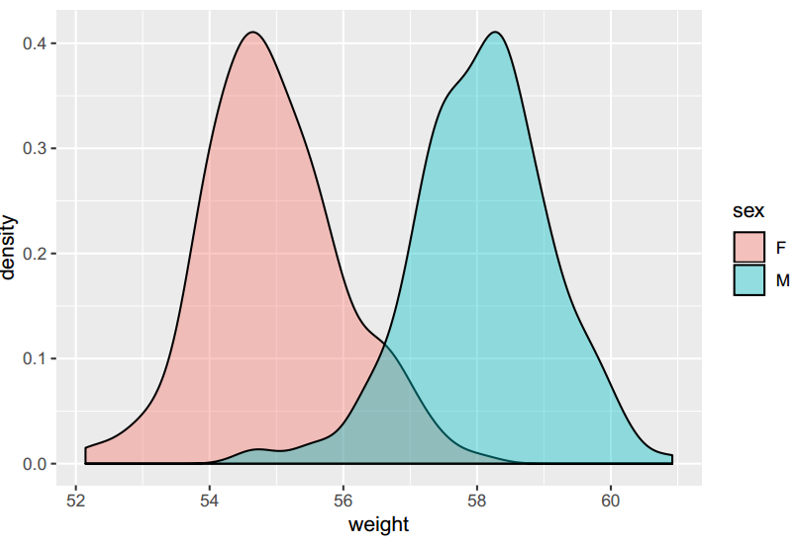到这里你已经可以画出比较高B格的density plot了，不过有些同学可能有 特殊的要求，比如把两组的平均值画出来，那本小仙再小秀一下?1.准备平均值数据并读取 mean<-read.csv(“your file path”) 2.画图 p+ geom_density(aes(color = sex), alpha=0.4)+ geom_vline(data = mean, aes(xintercept = mean, color=sex),linetype=“dashed”) #注释:前面是和之前一样作图语句，在后面直接加上这一句就好啦，xintercept表示x轴截断线，dashed表示是虚线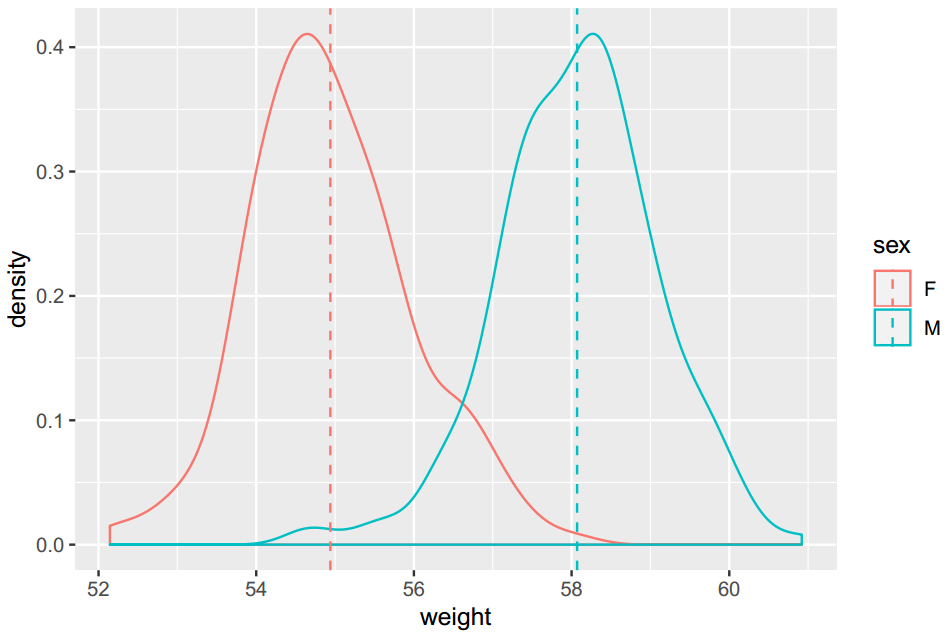好啦，今天的分享就到这里了，希望可以帮助到大家。有什么新的作图要求，可以留言给我哦！
导出高清图的方法在这里： R语言作图技巧——导出高清图 R语言作图系列还有： R语言作图——Histogram R语言作图——Violin plot R语言作图——Facet violin plot R语言作图——Line plot R语言作图——Density plot R语言作图——Bubble matrix R语言作图——Radar chart R语言作图——Pie chart
（公众号：生信了）展开全文作图 ggplot2
• 一份文件是介绍用R语言中如何利用核函数估计密度函数及其各阶导数的方法，是比较新的文献，2015年的，里面介绍了7种窗宽的确定方法以及R程序
• 二元变量绘制方法指南，点密度图和密度曲线可视化经典教程
• 1.核密度图 plot()函数，polygon()函数 d<- density(mtcars$mpg) class(d) plot(d) # polygon()函数根据顶点的x和y坐标（本例中由density()函数提供）绘制了多边形。 polygon(d,col='red',border='blue') rug... 核密度估计是用于估计随机变量概率密度函数的一种非参数方法。 1. 核密度图 plot()函数，polygon()函数 d<- density(mtcars$mpg)
class(d)
plot(d)

# polygon()函数根据顶点的x和y坐标（本例中由density()函数提供）绘制了多边形。
polygon(d,col='red',border='blue')
rug(mtcars$mpg,col='brown') 2. 可比较的核密度图 sm包中的sm.density.compare()函数 # install.packages('sm') library(sm) attach(mtcars) cyl.f <- factor(mtcars$cyl,labels=paste(c(4,6,8),"cylinder"))
colfill <- c(2:length(levels(cyl.f)))
sm.density.compare(mpg,cyl,xlable='Miles Per Gallon',
col=c("red","blue","green"))
title(main="MPG Distribution by Car Cylinders")
legend('topright',levels(cyl.f),fill=c("red","blue","green"))
# locator(1) 添加图例到鼠标点击的位置
# legend(locator(1),levels(cyl.f),fill=colfill)
detach(mtcars)

展开全文• R语言绘制核密度图实战（Kernel Density Plot） 目录 R语言绘制核密度图实战（Kernel Density Plot） #仿真数据 #修改核密度图的标题和轴标签 # 多边形函数为密度图着色 #为密度图添加均值竖线 #密度图和...
R语言绘制核密度图实战（Kernel Density Plot）

目录
R语言绘制核密度图实战（Kernel Density Plot）
#仿真数据

展开全文数据挖掘 机器学习 自然语言处理 人工智能
• 当数据点重叠很严重时，用散点图来观察变量关系就显得“力不从心”，这时就需要使用高密度图来显示双变量之间关系。 示例 #设置数据 set.seed(1234) n <- 10000 c1 <- matrix(rnorm(n,mean = 0,sd=0.5),ncol=2...
• 如果想要研究某一变量的分布情况，则需要使用直方密度曲线。 函数hist（x，breaks＝，）绘制直方。 x：是由一个数据值组成的数值向量 breaks：用于控制组的数量,breaks=seq(220,280,3)从220开始到280，...
• 直方图、概率密度图、散点图、箱线图函数和表示。 直方图 概率密度图 散点图 箱线图
• R语言绘制二维密度图 二维密度图显示了两个数值变量之间的关系，一个在x轴上表示，另一个在Y轴上表示，与散点图类似，然后计算二维空间中特定区域内的观测数，并用颜色梯度表示。二维密度图有几种类型，以下主要展示...
• 内容来源： http://lmdvr.r-forge.r-project.org/figures/figures.html 本文只是根据其代码作修改和加注释，希望能帮助大家学习R语言！！可视化
• 求取坐标点为绘制密度图做准备： zbd2008(csl2008) 加载包： library(lattice) 绘制直方图： histogram(csl$X2008,breaks=10) 添加需要的密度图： lines(zbd2008) 到此时，出现如下错误问题： 执行lines... • ## R语言密度曲线图 万次阅读 2018-01-11 15:00:11 密度曲线 ggplot(pp,aes(x=pp$FoldEnrichment,fill=new,alpha = 1/10))+geom_density()
• 本文只是根据其代码作修改和加注释，希望能帮助大家接触R学习！！ 以下是数据输入的过程 后面的代码块可以随意拼接，但这个部分一定要有！！ data(Chem97, package = "mlmRev") library("lattice") xtabs 作用相当...可视化
• R语言--制作group1和group2的分布并输出tiff格式300dpi保存 制作分布我选择的是制作直方+概率密度曲线~ ggplot(data,aes(value))+ #使用facet_grid()函数以group为类左右排列 facet_grid(group~.)+ #...
• y2,lwd=1.6,type="l") > lines(x1,y1,lwd=1.6,type="l",col="blue") > abline(v=0) > dev.off() F分布概率密度图 `R x1 (0,6,0.05) y1 (x1,10,25) y2 (x1,10,5) png("D:/Rwork/Picture/F分布.png",width=760,...机器学习
• 关于基本的直方设置、纵坐标对数变换以及基本的颜色设置，可以参考R语言ggplot2直方设置。 本文使用的数据为：R语言自带的mtcars数据集的mpg变量。以下内容中将首先给出目标图片和代码，并逐一解释。欢迎批评...ggplot2 数据分析
• 4.2.1直方 hist()的调用格式 hist(x,breaks="Sturges",freq=NULL,probability=!freq,col= NULL， main=paste("Histogram of" ,xname),xlim=range(breaks),ylim = NULL， xlab = xname， ylab， axes = TRUE， ...代码 统计分析
• 得出下面得密度图： 任务说明 给出相应得密度函数，还原上述图片，并给出置信区间。 1、案例 1.1代码 1.1.1包的载入以及密度函数的书写 library(ggplot2) # 载入包 fun <- function(Theta, Thetahat = 0.5, n = ...ggplot2
• ## R之核密度图

万次阅读 2017-12-23 20:22:50
# 密度图不失为一种用来观察连续型变量分布的有效方法。绘制密度图的方法（不叠加到另一幅图 # 上方）为： plot(density(x)) # # 其中的x是一个数值型向量。由于plot()函数会创建一幅新的图形，所以要向一 # 幅...
• ## R-密度图

千次阅读 2018-02-17 10:08:15
函数density() plot(density(rnorm(1000)))
• 直方 实例：nutshell包的births2006.smpl数据集，包含了2006年美国出生人口的数据的10%样本，每一条记录有13个变量。使用数据集前，需通过install.packages(“nutshell”)安装并加载。 以美国出生人口数据为例，...
• ## R语言核密度估计

千次阅读 2020-03-11 09:39:59
密度估计是在概率论中用来估计未知的密度函数，属于非参数检验方法之一，由Rosenblatt (1955)和Emanuel Parzen(1962)提出，又名Parzen窗（Parzen window）。 假设我们有n个数X1-Xn,我们要计算某一个数X的概率密度...数据分析
• 诸如核密度估计（KDE）的平滑方法被用于控制用于计算每种疾病率的空间支持的群体基础。平滑程度由用户定义的参数（带宽或阈值）控制，该参数影响疾病的分辨率和计算的速率的可靠性。已经提出了用于自动选择平滑...
• 目录 前言 一、数据 二、使用步骤 1.引入库 2.数据处理 3.画图 结果： 前言 下载地图资源...之类的，要API,原本打算申请的，但是网络实在是进不去，所以支持国产，阿里云上也提供了地理矢量的下载，话不多说看链接 ...开发语言
• 图表名称： 条形图 直方图 折线图 面积图 散点图 气泡图 箱线图 二维数据密度图
• <p>r语言画二维正态分布概率密度曲线的代码是什么呀</p>有问必答...